# Lists of mathematics topics

This article itemizes the various lists of mathematics topics. Some of these lists link to hundreds of articles; some link only to a few. The template to the right includes links to alphabetical lists of all mathematical articles. This article brings together the same content organized in a manner better suited for browsing.

The purpose of this list is not similar to that of the Mathematics Subject Classification formulated by the American Mathematical Society. Many mathematics journals ask authors of research papers and expository articles to list subject codes from the Mathematics Subject Classification in their papers. The subject codes so listed are used by the two major reviewing databases, Mathematical Reviews and Zentralblatt MATH. This list has some items that would not fit in such a classification, such as list of exponential topics and list of factorial and binomial topics, which may surprise the reader with the diversity of their coverage.

## Areas of basic mathematics

These lists include topics typically taught in secondary education or in the first year of university.

As a rough guide this list is divided into pure and applied sections although in reality these branches are overlapping and intertwined.

### Pure mathematics

#### Algebra

Algebra includes the study of algebraic structures, which are sets and operations defined on these sets satisfying certain axioms. The field of algebra is further divided according to which structure is studied; for instance, group theory concerns an algebraic structure called group.

#### Calculus and analysis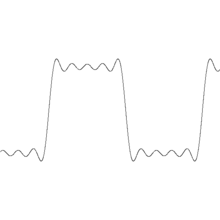Fourier series approximation of square wave in five steps.

Calculus studies the computation of limits, derivatives, and integrals of functions of real numbers, and in particular studies instantaneous rates of change. Analysis evolved from calculus.

#### Geometry and topology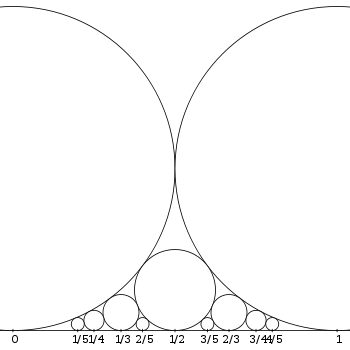Ford circles—A circle rests upon each fraction in lowest terms. Each touches its neighbors without crossing.

Geometry is initially the study of spatial figures like circles and cubes, though it has been generalized considerably. Topology developed from geometry; it looks at those properties that do not change even when the figures are deformed by stretching and bending, like dimension.

#### Combinatorics

Combinatorics concerns the study of discrete (and usually finite) objects. Aspects include "counting" the objects satisfying certain criteria (enumerative combinatorics), deciding when the criteria can be met, and constructing and analyzing objects meeting the criteria (as in combinatorial designs and matroid theory), finding "largest", "smallest", or "optimal" objects (extremal combinatorics and combinatorial optimization), and finding algebraic structures these objects may have (algebraic combinatorics).

#### Logic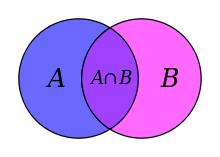Venn diagrams are illustrations of set theoretical, mathematical or logical relationships.

Logic is the foundation which underlies mathematical logic and the rest of mathematics. It tries to formalize valid reasoning. In particular, it attempts to define what constitutes a proof.

#### Number theory

Number theory studies the natural, or whole, numbers. One of the central concepts in number theory is that of the prime number, and there are many questions about primes that appear simple but whose resolution continues to elude mathematicians.

### Applied mathematics

#### Dynamical systems and differential equations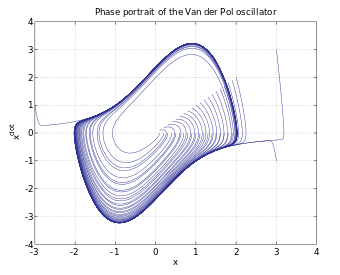Phase portrait of a continuous-time dynamical system, the Van der Pol oscillator.

A differential equation is an equation involving an unknown function and its derivatives.

In a dynamical system, a fixed rule describes the time dependence of a point in a geometrical space. The mathematical models used to describe the swinging of a clock pendulum, the flow of water in a pipe, or the number of fish each spring in a lake are examples of dynamical systems.

#### Mathematical physics

Mathematical physics is concerned with "the application of mathematics to problems in physics and the development of mathematical methods suitable for such applications and for the formulation of physical theories".1

#### Computation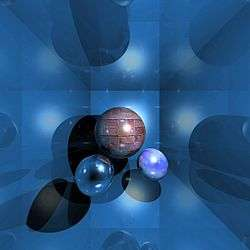Ray tracing is a process based on computational mathematics.

The fields of mathematics and computing intersect both in computer science, the study of algorithms and data structures, and in scientific computing, the study of algorithmic methods for solving problems in mathematics, science and engineering.

#### Information theory and signal processing

Information theory is a branch of applied mathematics and electrical engineering involving the quantification of information. Historically, information theory was developed to find fundamental limits on compressing and reliably communicating data.

Signal processing is the analysis, interpretation, and manipulation of signals. Signals of interest include sound, images, biological signals such as ECG, radar signals, and many others. Processing of such signals includes filtering, storage and reconstruction, separation of information from noise, compression, and feature extraction.

#### Probability and statistics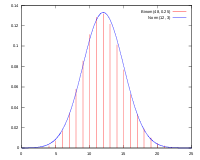The "bell curve"—the probability density function of the normal distribution.

Probability theory is the formalization and study of the mathematics of uncertain events or knowledge. The related field of mathematical statistics develops statistical theory with mathematics. Statistics, the science concerned with collecting and analyzing data, is an autonomous discipline (and not a subdiscipline of applied mathematics).

#### Game theory

Game theory is a branch of mathematics that uses models to study interactions with formalized incentive structures ("games"). It has applications in a variety of fields, including economics, evolutionary biology, political science, social psychology and military strategy.

#### Operations research

Operations research is the study and use of mathematical models, statistics and algorithms to aid in decision-making, typically with the goal of improving or optimizing performance of real-world systems.

## Mathematical statements

A mathematical statement amounts to a proposition or assertion of some mathematical fact, formula, or construction. Such statements include axioms and the theorems that may be proved from them, conjectures that may be unproven or even unprovable, and also algorithms for computing the answers to questions that can be expressed mathematically.

## Mathematical objects

Among mathematical objects are numbers, functions, sets, a great variety of things called "spaces" of one kind or another, algebraic structures such as rings, groups, or fields, and many other things.

## Equations named after people

### Mathematicians

Mathematicians study and research in all the different areas of mathematics. The publication of new discoveries in mathematics continues at an immense rate in hundreds of scientific journals, many of them devoted to mathematics and many devoted to subjects to which mathematics is applied (such as theoretical computer science and theoretical physics).

## Reference tables

### Integrals

In calculus, the integral of a function is a generalization of area, mass, volume, sum, and total. The following pages list the integrals of many different functions.

## Notes

• ^Note 1 : Definition from the Journal of Mathematical Physics .